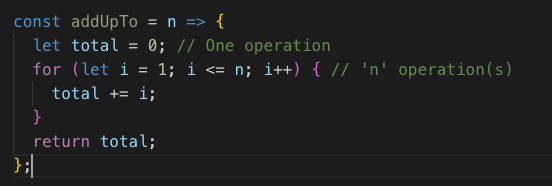# The Big O - An Introduction

Big O notation is a mathematical notation that describes the limiting behavior of a function when the argument tends towards a particular value or infinity. It is a member of a family of notations invented by Paul Bachmann, Edmund Landau, and others, collectively called Bachmann–Landau notation or asymptotic notation. - Wikipedia

### huh?

So what is the Big O and what is it used for? In short the simplest way to put it is that The Big-O is a mathematical expression used to determine how long it will take for an algorithm to run. In practice it is used to compare different functions that lead to the same end result but getting there using different approaches. > think performance <

It's common to come up with a solution to make the application work that isn't necessarily the best way of achieving the result. Let's talk about the most common types of Big O Notation

## Constant Time Algorithm - O(1)

With this type of algorithm no matter how complex the function may be, it will always take roughly the same amount of time to complete the operation.

`const addUpTo = n => (n * (n + 1)) / 2`

In this example we can see that regardless of what 'n' equals there will be 3 operations. Let's say that in this case n = 4.

`4 * (4 + 1) / 2`

This type of operation is always going to be ideal in terms of speed. It doesn't matter how big 'n' gets, this operation can be performed quickly

## Linear Time Algorithm - O(n)

This algorithm takes exponentially longer to complete the bigger 'n' gets. We can rewrite the code above to illustrate the point.

In this example we are creating another function that adds up to 'n'. Let's substitute the 'n' for 4 again and see what it looks likeAs we can see with the second operation, the for loop will continue to run for as many times as 'n' equals. This second operation is how most developers would originally come to the answer (as it is most logical to follow) but for performance reasons it doesn't scale very well. What if 'n' were to equal 1000000000000. That means the for loop would iterate over and over and over and over.... you get it. In short there are no set amount of operations and takes longer to complete the larger 'n' is.